# ISEE Lower Level Quantitative : Identifying Numbers

## Example Questions

← Previous 1 3 4 5 6 7 8 9 28 29

### Example Question #1 : Identifying Numbers

What number is one thousand?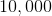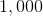Explanation:in word form is one thousand.

### Example Question #2 : Identifying Numbers

What number is one hundred?Explanation:in word form is one hundred.

### Example Question #3 : Identifying Numbers

What number is two hundred?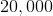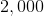Explanation:in word form is two hundred.

### Example Question #4 : Identifying Numbers

What number is nine hundred?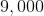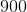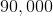Explanation:in word form is nine hundred.

### Example Question #5 : Identifying Numbers

What number is forty-three?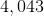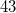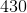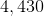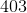Explanation:in word form is forty-three.

### Example Question #6 : Identifying Numbers

What number is eight hundred four?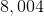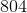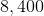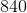Explanation:in word form is eight hundred forty-four.

### Example Question #7 : Identifying Numbers

What number is ninety-six?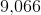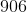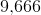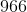Explanation:in word form is ninety-six.

### Example Question #8 : Identifying Numbers

What number is nine hundred forty-eight?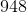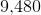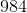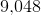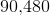Explanation:in word form is nine hundred forty-eight.

### Example Question #9 : Identifying Numbers

What number is nine hundred?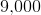Explanation:in word form is nine hundred.

### Example Question #10 : Identifying Numbers

What number is nine hundred thirty-six?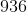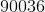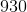in word form is nine hundred thirty-six.